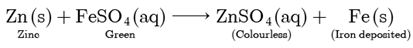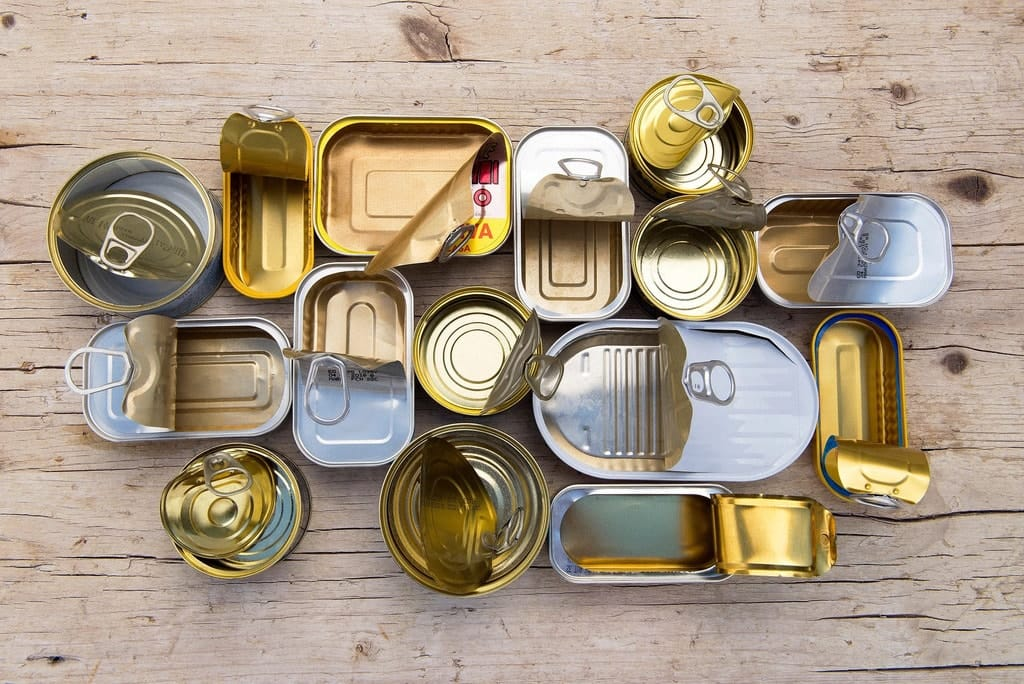Assertions & Reason Type Practice Questions: Metals & Non-metals

# Assertions & Reason Type Practice Questions: Metals & Non-metals - Science Class 10

Direction: In the following questions, a statement of assertion (A) is followed by a statement of reason (R). Mark the correct choice as:
Question 1:
Assertion: When zinc is added to a solution of iron (II) sulphate, no change is observed.
Reason: Zinc is less reactive than iron
(a) Both assertion (A) and reason (R) are true and reason (R) is the correct explanation of assertion (A).
(b) Both assertion (A) and reason (R) are true but reason (R) is not the correct explanation of assertion (A).
(c) Assertion (A) is true but reason (R) is false.
(d) Assertion (A) is false but reason (R) is true.
(e) Both Assertion and Reason are false.

Assertion (A) is false but reason (R) is true. Both Assertion and Reason are false. Zinc being more reactive than iron displaces iron from iron (II) sulphate solution.
Thus, the green colour of the solution fades and iron metal gets deposited.Question 2:
Assertion: Food cans are coated with tin and not with zinc.
Reason: Zinc is more reactive than tin.

(a) Both assertion (A) and reason (R) are true and reason (R) is the correct explanation of assertion (A).
(b) Both assertion (A) and reason (R) are true but reason (R) is not the correct explanation of assertion (A).
(c) Assertion (A) is true but reason (R) is false.
(d) Assertion (A) is false but reason (R) is true.
(e) Both Assertion and Reason are false.

Both assertion (A) and reason (R) are true and reason (R) is the correct explanation of assertion (A). Food cans are coated with tin not with zinc because zinc is more reactice than tin, it can react with organic acids present in food.Tin coated food cans

Question 3:
Assertion: Platinum, gold and silver are used to make jewellery.
Reason: Platinum, gold and silver are least reactive metals.
(a) Both assertion (A) and reason (R) are true and reason (R) is the correct explanation of assertion (A).
(b) Both assertion (A) and reason (R) are true but reason (R) is not the correct explanation of assertion (A).
(c) Assertion (A) is true but reason (R) is false.
(d) Assertion (A) is false but reason (R) is true.
(e) Both Assertion and Reason are false.

Both assertion (A) and reason (R) are true and reason (R) is the correct explanation of assertion(A). Platinum, gold and silver are highly malleable lustraus and least reactive, i.e. noble metals, so they are not corroded by air and water easily.

Question 4:
Assertion: Iron is found in the free state n nature.
Reason: Iron a highly reactive element.
(a) Both assertion (A) and reason (R) are true and reason (R) is the correct explanation of assertion (A).
(b) Both assertion (A) and reason (R) are true but reason (R) is not the correct explanation of assertion (A).
(c) Assertion (A) is true but reason (R) is false.
(d) Assertion (A) is false but reason (R) is true.
(e) Both Assertion and Reason are false.

Iron is found in free state in nature but is mildly reactive towards atmospheric oxidation.

Question 5:
Assertion: Carbon reacts with oxygen to form carbon dioxide which is an acidic oxide.
Reason: Non-metals form acidic oxides.
(a) Both assertion (A) and reason (R) are true and reason (R) is the correct explanation of assertion (A).
(b) Both assertion (A) and reason (R) are true but reason (R) is not the correct explanation of assertion (A).
(c) Assertion (A) is true but reason (R) is false.
(d) Assertion (A) is false but reason (R) is true.
(e) Both Assertion and Reason are false.

Both assertion (A) and reason (R) are true explanation of assertion (A). Carbon being a non-metal form acidic oxides, i.e., their aqueous solution turns blue litmus solution red.

Question 6:
Assertion: Coke and flux are used in smelting.
Reason: The phenomenon in which ore is mixed with suitable flux and coke is heated to fusion is known as smelting.
(a) Both assertion (A) and reason (R) are true and reason (R) is the correct explanation of assertion (A).
(b) Both assertion (A) and reason (R) are true but reason (R) is not the correct explanation of assertion (A).
(c) Assertion (A) is true but reason (R) is false.
(d) Assertion (A) is false but reason (R) is true.
(e) Both Assertion and Reason are false.

Both assertion (A) and reason (R) are true but reason (R) is not the correct explanation of assertion (A). Smelting is a process of applying heat to ore in order to extract a base metal. It is used to extract many metals from their ores, including silver, iron, copper, and other base metals.

Question 7:
Assertion: Leaching is a process of reduction.
Reason: Leaching involves treatment of the ore with a suitable reagent so as to make it solube while impurities remains insoluble.

(a) Both assertion (A) and reason (R) are true and reason (R) is the correct explanation of assertion (A).
(b) Both assertion (A) and reason (R) are true but reason (R) is not the correct explanation of assertion (A).
(c) Assertion (A) is true but reason (R) is false.
(d) Assertion (A) is false but reason (R) is true.
(e) Both Assertion and Reason are false.

Leaching is the process of concentration of ores in which metal is made soluble in a solvent while the impurities remain insoluble and get separated out. It does not involve any redox reaction.

Question 8:
Assertion: Lead, tin and bismuth are purified by liquation method.
Reason: Lead, tin and bismuth have low m.p. as compared to impurities.
(a) Both assertion (A) and reason (R) are true and reason (R) is the correct explanation of assertion (A).
(b) Both assertion (A) and reason (R) are true but reason (R) is not the correct explanation of assertion (A).
(c) Assertion (A) is true but reason (R) is false.
(d) Assertion (A) is false but reason (R) is true.
(e) Both Assertion and Reason are false.

Liquation process is used when the impurity is less fusible than the metal itself. lead, tin and bismuth have low m.p. as compared to impurities.

Hence, lead, tin and bismuth are purified by liquation method.

Question 9:

Assertion: Leaching is a process of reduction.
Reason: Leaching involves treatment of the ore with a suitable reagent so as to make it soluble while impurities remains insoluble.

(a) Both assertion (A) and reason (R) are true and reason (R) is the correct explanation of assertion (A).
(b) Both assertion (A) and reason (R) are true but reason (R) is not the correct explanation of assertion (A).
(c) Assertion (A) is true but reason (R) is false.
(d) Assertion (A) is false but reason (R) is true.
(e) Both Assertion and Reason are false.

Assertion (A) is false but reason (R) is true. Leaching is a process where ore is soluble and impurities are insoluble, widely used extractive metallurgy technique which converts metals into soluble salts in aqueous media.

Question 10:
Assertion: Levigation is used for the separation of oxide ores from impurities.
Reason: Ore particles are removed by washing in a current of water.
(a) Both assertion (A) and reason (R) are true and reason (R) is the correct explanation of assertion (A).
(b) Both assertion (A) and reason (R) are true but reason (R) is not the correct explanation of assertion (A).
(c) Assertion (A) is true but reason (R) is false.
(d) Assertion (A) is false but reason (R) is true.
(e) Both Assertion and Reason are false.

Assertion (A) is true but reason (R) is false. Levigation method is commonly used for oxide ores such as haematite, tin stone and native ores of Au, Ag, etc.

The document Assertions & Reason Type Practice Questions: Metals & Non-metals | Science Class 10 is a part of the Class 10 Course Science Class 10.
All you need of Class 10 at this link: Class 10

## Science Class 10

78 videos|509 docs|153 tests

## FAQs on Assertions & Reason Type Practice Questions: Metals & Non-metals - Science Class 10

 1. What are metals and non-metals?Ans. Metals are elements that are typically shiny, malleable, and good conductors of heat and electricity. They are usually solid at room temperature, except for mercury which is a liquid. Non-metals, on the other hand, are elements that lack metallic properties. They are generally poor conductors of heat and electricity and can exist in all three states of matter.
 2. What are some examples of metals and non-metals?Ans. Examples of metals include iron, copper, aluminum, gold, and silver. Non-metal examples include carbon, oxygen, nitrogen, sulfur, and chlorine.
 3. What are the physical properties of metals?Ans. Metals have several physical properties, such as being malleable (can be hammered into thin sheets), ductile (can be drawn into wires), and having high melting and boiling points. They are also generally dense, shiny, and good conductors of heat and electricity.
 4. What are the physical properties of non-metals?Ans. Non-metals have physical properties that are opposite to those of metals. They are generally brittle and cannot be hammered or drawn into wires. They have low melting and boiling points and are poor conductors of heat and electricity. Non-metals are usually dull and have lower densities compared to metals.
 5. How do metals and non-metals react with acids?Ans. Metals react with acids to produce hydrogen gas and a salt. For example, when zinc reacts with hydrochloric acid, it produces zinc chloride and hydrogen gas. Non-metals, however, do not react with acids.

## Science Class 10

78 videos|509 docs|153 testsExplore Courses for Class 10 examSignup to see your scores go up within 7 days! Learn & Practice with 1000+ FREE Notes, Videos & Tests.
10M+ students study on EduRev
Track your progress, build streaks, highlight & save important lessons and more!
Related Searches

,

,

,

,

,

,

,

,

,

,

,

,

,

,

,

,

,

,

,

,

,

;